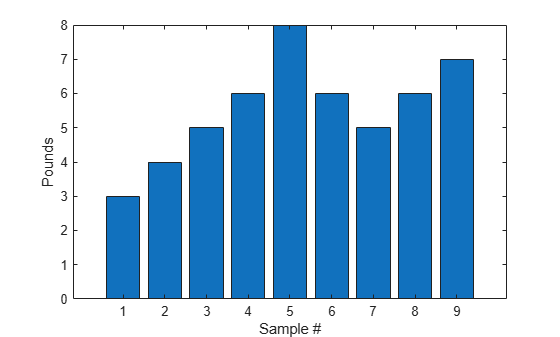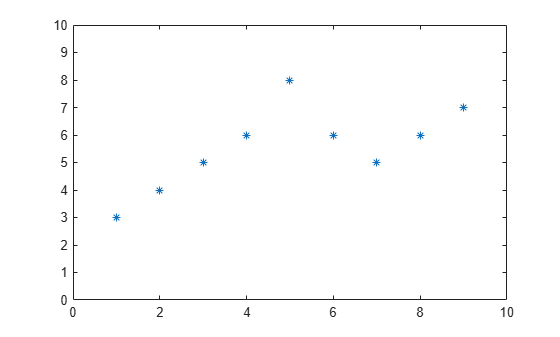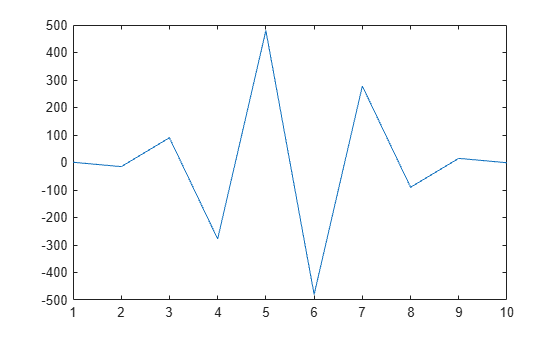# Basic Matrix Operations

This example shows basic techniques and functions for working with matrices in the MATLAB® language.

First, let's create a simple vector with 9 elements called `a`.

`a = [1 2 3 4 6 4 3 4 5]`
```a = 1×9 1 2 3 4 6 4 3 4 5 ```

Now let's add 2 to each element of our vector, `a`, and store the result in a new vector.

Notice how MATLAB requires no special handling of vector or matrix math.

`b = a + 2`
```b = 1×9 3 4 5 6 8 6 5 6 7 ```

Creating graphs in MATLAB is as easy as one command. Let's plot the result of our vector addition with grid lines.

```plot(b) grid on```MATLAB can make other graph types as well, with axis labels.

```bar(b) xlabel('Sample #') ylabel('Pounds')```MATLAB can use symbols in plots as well. Here is an example using stars to mark the points. MATLAB offers a variety of other symbols and line types.

```plot(b,'*') axis([0 10 0 10])```One area in which MATLAB excels is matrix computation.

Creating a matrix is as easy as making a vector, using semicolons (;) to separate the rows of a matrix.

`A = [1 2 0; 2 5 -1; 4 10 -1]`
```A = 3×3 1 2 0 2 5 -1 4 10 -1 ```

We can easily find the transpose of the matrix `A`.

`B = A'`
```B = 3×3 1 2 4 2 5 10 0 -1 -1 ```

Now let's multiply these two matrices together.

Note again that MATLAB doesn't require you to deal with matrices as a collection of numbers. MATLAB knows when you are dealing with matrices and adjusts your calculations accordingly.

`C = A * B`
```C = 3×3 5 12 24 12 30 59 24 59 117 ```

Instead of doing a matrix multiply, we can multiply the corresponding elements of two matrices or vectors using the .* operator.

`C = A .* B`
```C = 3×3 1 4 0 4 25 -10 0 -10 1 ```

Let's use the matrix A to solve the equation, A*x = b. We do this by using the \ (backslash) operator.

`b = [1;3;5]`
```b = 3×1 1 3 5 ```
`x = A\b`
```x = 3×1 1 0 -1 ```

Now we can show that A*x is equal to b.

`r = A*x - b`
```r = 3×1 0 0 0 ```

MATLAB has functions for nearly every type of common matrix calculation.

There are functions to obtain eigenvalues ...

`eig(A)`
```ans = 3×1 3.7321 0.2679 1.0000 ```

... as well as the singular values.

`svd(A)`
```ans = 3×1 12.3171 0.5149 0.1577 ```

The "poly" function generates a vector containing the coefficients of the characteristic polynomial.

The characteristic polynomial of a matrix `A` is

`$det\left(\lambda I-A\right)$`

`p = round(poly(A))`
```p = 1×4 1 -5 5 -1 ```

We can easily find the roots of a polynomial using the `roots` function.

These are actually the eigenvalues of the original matrix.

`roots(p)`
```ans = 3×1 3.7321 1.0000 0.2679 ```

MATLAB has many applications beyond just matrix computation.

To convolve two vectors ...

`q = conv(p,p)`
```q = 1×7 1 -10 35 -52 35 -10 1 ```

... or convolve again and plot the result.

`r = conv(p,q)`
```r = 1×10 1 -15 90 -278 480 -480 278 -90 15 -1 ```
`plot(r);`At any time, we can get a listing of the variables we have stored in memory using the `who` or `whos` command.

`whos`
``` Name Size Bytes Class Attributes A 3x3 72 double B 3x3 72 double C 3x3 72 double a 1x9 72 double ans 3x1 24 double b 3x1 24 double p 1x4 32 double q 1x7 56 double r 1x10 80 double x 3x1 24 double ```

You can get the value of a particular variable by typing its name.

`A`
```A = 3×3 1 2 0 2 5 -1 4 10 -1 ```

You can have more than one statement on a single line by separating each statement with commas or semicolons.

If you don't assign a variable to store the result of an operation, the result is stored in a temporary variable called `ans`.

`sqrt(-1)`
```ans = 0.0000 + 1.0000i ```

As you can see, MATLAB easily deals with complex numbers in its calculations.